﻿ Analytical Solution of Homogeneous Damped Mathieu Equation

Analytical Solution of Homogeneous Damped Mathieu Equation

Dmitri Yerchuck, Alla Dovlatova, Yauhen Yerchak, Felix BorovikOPEN ACCESSPEER-REVIEWED

Analytical Solution of Homogeneous Damped Mathieu Equation

Dmitri Yerchuck1,, Alla Dovlatova2, Yauhen Yerchak3, Felix Borovik1

1Heat-Mass Transfer Institute of National Academy of Sciences of RB, Brovka Str., 15, Minsk, RB

2M.V.Lomonosov Moscow State University, Moscow

3Belarusian State University, Nezavisimosti Avenue 4, Minsk, RB

Abstract

The general solution of the homogeneous damped Mathieu equation in the analytical form, allowing its practical using in many applications, including superconductivity studies, without numerical calculations has been found.

• Yerchuck, Dmitri, et al. "Analytical Solution of Homogeneous Damped Mathieu Equation." International Journal of Physics 2.5 (2014): 165-169.
• Yerchuck, D. , Dovlatova, A. , Yerchak, Y. , & Borovik, F. (2014). Analytical Solution of Homogeneous Damped Mathieu Equation. International Journal of Physics, 2(5), 165-169.
• Yerchuck, Dmitri, Alla Dovlatova, Yauhen Yerchak, and Felix Borovik. "Analytical Solution of Homogeneous Damped Mathieu Equation." International Journal of Physics 2, no. 5 (2014): 165-169.

 Import into BibTeX Import into EndNote Import into RefMan Import into RefWorks

1. Introduction and Background

It is known, that a number of physical phenomena can be described mathematically by Mathieu equation. For instance, the equation of the motion for the flux lattice in the superconductor theory is the following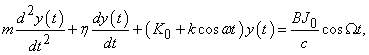(1)

where B is the magnetic induction in the sample, m is the total mass (per unit length) of the flux lattice,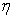is the viscosity coefficient,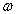is the frequency of the modulating magnetic field, c is the light velocity, J0 and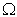are the amplitude and the frequency of the microwave current, respectively, K0 and k are amplitudes of constant component and an alternating component in the function K(t) in the relationship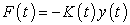(2)

between the force F(t) and small displacement y(t) of the flux lattice from its equilibrium position, that is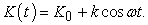(3)

The equation (1) is the inhomogeneous damped Mathieu equation.

The Mathieu equation is well knowm in the theory of differential equations, see for example [2, 3, 4, 5].

At that, the so-called general Mathieu equation is the following equation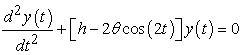(4)

where h and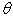are real or complex constants. The known solution of the general Mathieu equation (4) is built in the form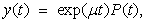(5)

where P(t) is a periodical function with the period, equal to π, μ is so-called characteristic index, depending on the values of h and θ. The function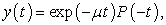(6)

represents itself the second solution. The solutions (5) and (6) are linearly independent, they produce the fundamental system of the solutions, excluding the case, when iμ∈Z, that is, to the set of whole numbers . Further, the solution (5) is written formally in the form of the following infinite series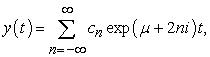(7)

in which for the set of coefficients {cn} the following recurrent relations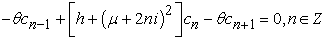(8)

were obtained. It is the only algorithm for the numerical solution. In fact, the analytical solution of the general Mathieu equation (4) was not found. The existence the only algorithn for the numerical solution is inconveniently for the practical usage of Mathieu equation, especially in the cases of physical applications when analytical dependences are required to understand the physical processes. For instance, the authors of the work  have preferred instead of trying to solve Mathieu equation numerically to use the corresponding linearized equation.

Let us remark, that an (in) homogeneous damped Mathieu equation can be reduced to an (in) homogeneous general Mathieu equation by the following transformation of the function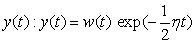.

At the same time, the authors of  remark, that when static magnetic field H becomes too large [larger than 800 G], the flux structure becomes probably too complex for the simple model proposed to remain valid.

It is clear on the given example, that an analytical solution of the Mathieu equation remains to be very actual for its physical science applications and for engineering.

On the other hand, an analytical solution of the Mathieu equation has also the mathematical theoretical aspect. It is determined by the fact that the solution of a number of differential equations is reduced to the solution of the Mathieu equation. They, for example, are

1)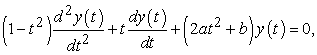(9)

The transformation of variable t = cosz leads to the Mathieu equation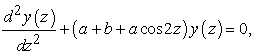(10)

2)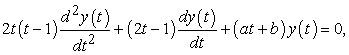(11)

The transformation of variable t = cos2z leads to the Mathieu equation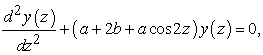(12)

3)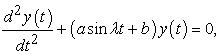(13)

The transformation of variable,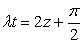leads to the Mathieu equation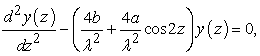(14)

4)

The equations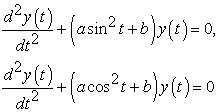(15)

are transformed to Mathieu equations by using of trigonometric formulae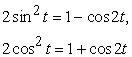(16)

correspondingly.

The aim of the given work is to find the analytical solution of the general Mathieu equation.

2. Results

Theorem. The general solution of the general Mathieu equation can be represented analytically to be the superposition of Bessel functions of the first and the second kinds.

Proof.

It is evident, that the equation (1) can be represented in the form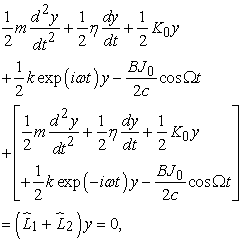(17)

where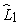and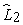are differential operators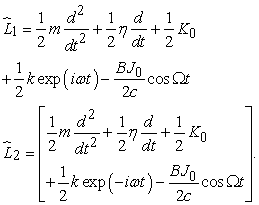(18)

It is also evident that the partial solution of the starting equation will correspond to the intersection of sets of the solutions satisfying simultaneously to the equations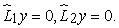(19)

So, we have to solve the equations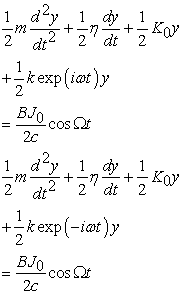(20)

or in the equivalent form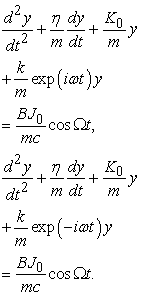(21)

The case(22)

can be also studied. There are a number of variants to simplify the solution of (22) using the symmetry of y(t). In the case, if y(t) is even,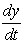is uneven,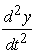is even from (22) we obtain by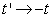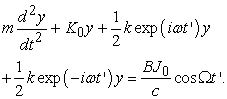(23)

Given case is equivalent to the task above formulated by the relations (17) - (19). Further, if y(t) is even,is uneven,is even from (22) we obtain by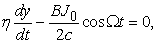(24)

that is the simple differential equation of the first order, the solution of which is evident.

The homogeneous equations, corresponding to inhomogeneous equations (1), that is, the equations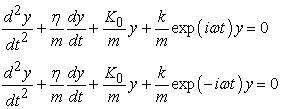(25)

can be solved strictly. Let us designate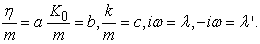Then, according to , the solutions are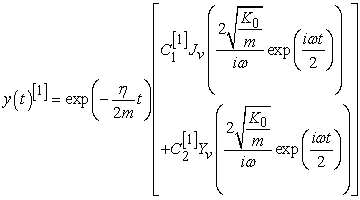(26)

for the first equation in (25) and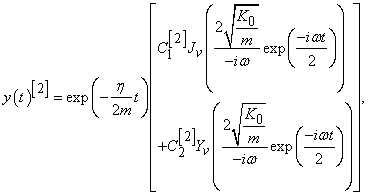(27)

for the second equation in (25). Here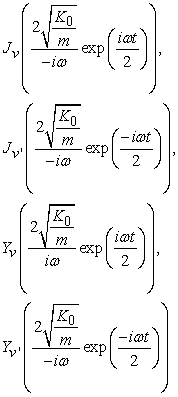(28)

are Bessel functions of the first kind and of the second kind of index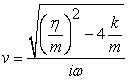(29)

for the equation (28) and of index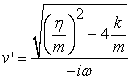(30)

for the equation (29).

Then the set of functions, satisfying to the relation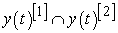(31)

will be the solution of the homogeneous equation, corresponding to the starting inhomogeneous equation (1). Given conclusion is correct, if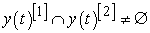. In particular, the relationis satisfied for the case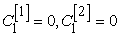and by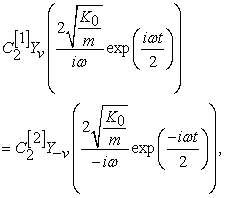(32)

that is, in the case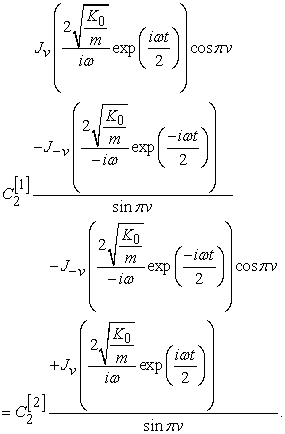(33)

Hence we obtain the conditions, by which the relation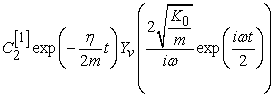(34)

will be the solution of the homogeneous damped Mathieu equation. They are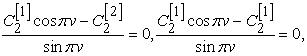(35)

that is, by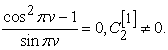(36)

Consequently, vZ, that is v has to belong to the set of whole numbers.

Therefore, the relation (34) is the solution of homogeneous damped Mathieu equation by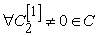and by vZ. It means, that there are restrictions on possible values of parameters in the starting homogeneous damped Mathieu equation. They are the following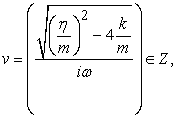(37)

that is,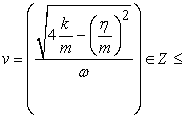(38)

It is evident that by= 0 we obtain the solution of the homogeneous undammed Mathieu equation, that is, the solution of the general Mathieu equation.

To obtain the fundamental system of the solutions of the homogeneous damped Mathieu equation, we have to find the second linearly independent solution. It can be easily done, if to take into account, that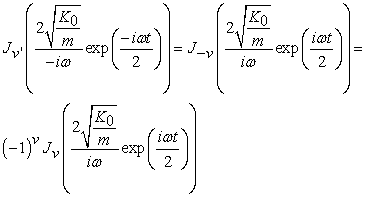(39)

for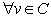.

Since the replacement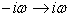is equivalent to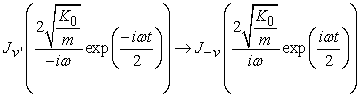(40)

we obtain, that bythe expression (31) will be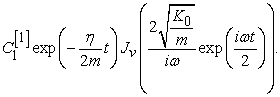(41)

The given expression is the second linearly independent solution of the homogeneous damped Mathieu equation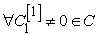and byat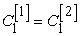for even vZ and at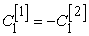for uneven vZ, that is at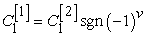. Its linear independence from the first solution follows from linear independence of Bessel functions on the first and the second kinds. At fixed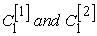the relations (34) and (41) represent themselves the fundamental system of the solution of the homogeneous damped Mathieu equation. So, the general solution of the homogeneous damped Mathieu equation is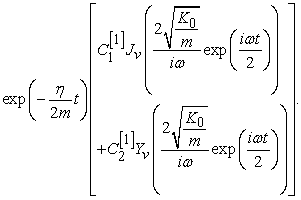(42)

The theorem is proved.

Thus, we have found the general solution of the homogeneous damped Mathieu equation in the analytical form, allowing its practical using in many applications without numerical calculations, since Bessel functions are well known. The solution of the inhomogeneous damped Mathieu equation can be obtained by the expansion of the general solution of the homogeneous damped Mathieu equation in Fourier series.

Reference

  Kim P W, de Graaf A M, Chen J T, Friedman E J, and Kim S H,Phase Reversal and Modulated Flux Motion in Superconducting Thin Films, Phys. Rev. B, 6, N 3 (1972) 887-893.In article  Kotowski G, Z.Angew. Math. Mech., 23 (1943) 213.In article  Meixner J and SchÄafke F W, Mathieusche Funktionen und SphÄaroidfunktionen, Springer, Berlin, 1954.In article  Bateman H,Erdelyi A, Higher Transcendental Functions, V.3, New York, Toronto, London, MC Graw-Hill Book Company, Inc., 1955, M., Nauka, 1967, 300 pp.In article  Mac Lachlan, Theory and Applications of Mathieu Functions, M., Izd.In.Lit., 1953, 476 pp.In article  Zaitsev V F, Polyanin A D, Hand-Book on Ordinary Differential Equations, M, Fizmatlit, 2001, 576 pp.In article